# Right triangle - 9th grade (14y) - math problems

1. CosineThe point (8, 6) is on the terminal side of angle θ. cos θ = ?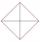In the square ABCD point P is in the middle of the DC side and point Q in the middle pages AD. If the area of quadrilateral BQPC is 49 cm2, what is the area of ABCD?
3. Cone and the ratioRotational cone has a height 23 cm and the ratio of the base surface to lateral surface is 7: 9. Calculate a surface of the base and the lateral surface.
4. SailsWe known heights 220, 165 and 132 of sail. It has triangular shape. What is the surface of the sail?
5. RT 11Calculate the area of right tirangle if its perimeter is p = 45 m and one cathethus is 20 m long.
6. DistanceWha is the distance between the origin and the point (18; 22)?
7. Leg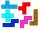Determine the area of a trapezoid with bases 71 and 42 and height is 4 shorter than the its leg.
8. CosineCalculate the cosine of the smallest internal angle in a right-angled triangle with cathetus 3 and 8 and with the hypotenuse 8.544.
9. Inscribed rectangle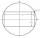The circle area is 216. Determine the area of inscribed rectangle with one side 5 long.
10. ISO trapeziumCalculate area of isosceles trapezoid with base 95 long, leg 27 long and with the angle between the base and leg 70 degrees.
11. 30-60-90The longer leg of a 30°-60°-90° triangle measures 5. What is the length of the shorter leg?
12. Chord - TSThe radius of circle k measures 68 cm. Arc GH = 47 cm. What is TS?
13. EQL triangle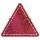Calculate inradius and circumradius of equilateral triangle with side a=77 cm.
14. FlowerbedFlowerbed has the shape of an isosceles obtuse triangle. Arm has a size 5.5 meters and an angle opposite to the base size is 94°. What is the distance from the base to opposite vertex?
15. Semicircle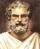In the semicircle with center S and the diameter AB is constructed equilateral triangle SBC. What is the magnitude of the angle ∠SAC?
16. Cable car 2Cable car rises at an angle 41° and connects the upper and lower station with an altitude difference of 1175 m. How long is the track of cable car?
17. Circle sectionEquilateral triangle with side 33 is inscribed circle section whose center is in one of the vertices of the triangle and the arc touches the opposite side. Calculate: a) the length of the arc b) the ratio betewwn the circumference to the circle sector
18. Trapezoid ABCD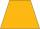Calculate the perimeter of trapezoid ABCD if we know the side c=15, b=19 which is also a height and side d=20.
19. Hexagon ACalculate area of regular hexagon inscribed in circle with radius r=9 cm.
20. TowerThe top of the tower is a regular hexagonal pyramid with base edge 8 meters long and a height 5 meters. How many m2 of the sheet is required to cover the top of the tower if we count 8% of the sheet waste?

Do you have an interesting mathematical word problem that you can't solve it? Enter it, and we can try to solve it.

We will send a solution to your e-mail address. Solved examples are also published here. Please enter the e-mail correctly and check whether you don't have a full mailbox.

Please do not submit problems from current active competitions such as Mathematical Olympiad, correspondence seminars etc...